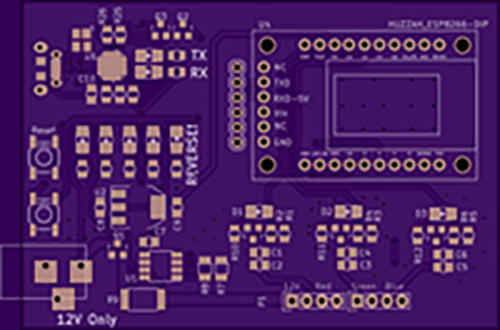﻿ Measure PWM Current with a Modified Moving Average | EngineersGarage

# Measure PWM Current with a Modified Moving Average

### Submitted By:

Shalini Verma

As a techie, we do understand the role of Pulse with modulation (PWM) in our daily life as well as in scientific life. From controlling lights to setting speed of motors to the advanced generation of analog reference voltages, their usage is diverse but accompanies a special challenge while measuring the voltage or current of a PWM signal. James Lewis, through this tutorial, has perfectly explained how to measure PWM current with a modified moving average (MMA) while including INA219 current sensor on the LED power supply.The current sensor used in this project is fast and precise and works by measuring the voltage drop across a precision shunt resistor that the load current flows through. Though the measurement was fast and was providing prompt answers. But it provided a random value; each time the sensor was checked and hence appeared as a major problem.

The result after sampling the waveform was in different forms and transitions, where only high was considered useful. Moreover there were no stable values and it was consuming hundreds of bytes of RAM just to get an idea of the RMS current. In order to calculate power usage easily, Modified Moving Average (MMA) was used that gave more accurate results with less consumption of RAM ad is compatible to Uno’s ATmega328p.

The finest advantage of using MMA is that it requires knowing three values only i.e. “Moving Average Sum,” “Moving Average,” and the number of samples. The former value i.e. Moving Average Sum is the previous measurements summed together, with a slight change. The number of samples can be a constant, saving a byte or two of memory.

In the later value i.e. Moving Average, a new sample is added to the revised moving average and a new average value is calculated. MMA can be used for determining the average output value when measuring PWM Current or PWM Voltage. When calculating power, this is helpful because it saves time. When looking at a voltage, you can get an idea of the effective voltage for a load like a LED or Motor.

For the codes of this project as well as for better understanding of MMA, visit the following link.